﻿﻿ Inductor with Superimposed DC (Minor Loop Permeability)examples｜products｜Murata Software Co., Ltd.# Example28Inductor with Superimposed DC (Minor Loop Permeability)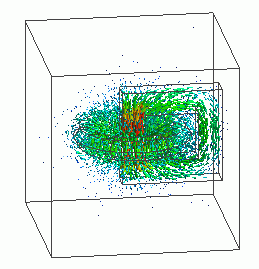General

• The inductance of an inductor with superimposed DC is analyzed.

• The minor-loop permeability is used.

• This is a static analysis. The high-frequency characteristics such as skin effect are not taken into account.
See Exercise 36 for high-frequency analysis.

• The vectors of the magnetic field and the magnetic flux density are solved.

• Unless specified in the list below, the default conditions will be applied.

### Analysis Space

 Item Settings Analysis Space 3D Model unit mm

### Analysis Conditions

 Item Settings Solvers Magnetic Field Analysis [Gauss] Analysis Type Static analysis Options N/A

### Model

A loop coil (coil) and a magnetic core (core) with gap are placed.

As the magnetic field concentrates in the gap of the magnetic core, small mesh size is applied (0.2mm).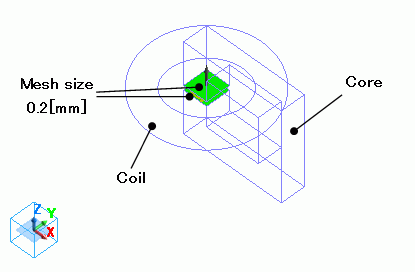### Body Attributes and Materials

 Body Number/Type Body Attribute Name Material Name Mesh Size 1/Solid Coil 008_Cu * 0.5 9/Solid NonL NonL_mu0_2 0.5

* Available from the Material DB

Nonlinear material is defined on the nonlinearity table.

To solve the inductance under DC-biased condition, the magnetic field is solved first with the major loop.
Then, by using the minor loop and this magnetic field, the permeability is obtained for the inductance calculation.

Material Name

Tab

Settings

NonL_mu0_2

Permeability

Magnetization Characteristic Type: Select B-H curve

Select “Use the minor-loop permeability”

B-H Curve Table

 Magnetic Field [A/m] Magnetic Flux Density [T] 0 0x10^-4 1 2×10^-4 2 5×10^-4 10 7×10^-4

This B-H curve is for DC bias.
It is called Major Loop.

Permeability

for Minor Loop

Magnetization Characteristic Type: Select B-H curve

B-H Curve Table

 Magnetic Field [A/m] Magnetic Flux Density [T] 0 0x10^-4 1 1×10^-4 2 2.5×10^-4 10 3.5×10^-4

This B-H curve is for AC operation.

Body attribute is set up as follows to apply current to the coil.

Enter the direct current value.

AC current cannot be given. It is assumed to be significantly small compared to DC current.

 Body Attribute Name Tab Settings Coil Current Waveform: Constant Current: 1[A] Turns: 1[Turns] Direction: Loop Coil/Magnetic Field Direction Magnetic Field Vector: X=0, Y=0, Z=1

No setting.

### Results

To see the results of inductance calculation, go to the [Results] tabclick [Table]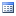.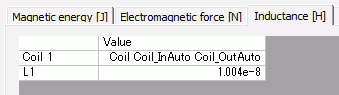The vectors of the magnetic flux density are shown below.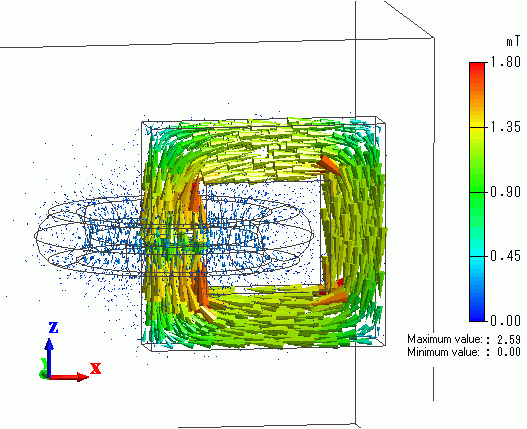The figure below shows the inductance when “Use the minor-loop permeability” is deselected for NonL_mu0_2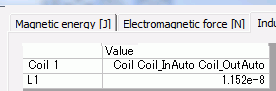It is greater than the value calculated with minor-loop permeability.

This indicates the tendency that the major-loop slope is greater than the minor-loop slope.

﻿ ﻿